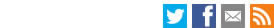Search the LSE website# Abstract:CEP discussion paper
Ex Post Versus Ex Ante Measures of the User Cost of Capital
Nicholas Oulton
July 2005
Paper No' CEPDP0698:
Full PaperJEL Classification: O470; E010; C430; E220

When doing growth accounting, should we use ex post or ex ante measures of user costs to calculate the contribution of capital? The answer, based on a simple model of temporary equilibrium, is that ex post is better in theory. In practice researchers usually calculate ex post user costs by assuming that the rate of return is equalised across assets. But this is only true if expectations are correct. A numerical example shows that either ex ante or ex post can be closer to the true measure, depending on the parameters. I propose a hybrid method that makes use of elements of both approaches. I test this and the other methods using data for 31 UK industries.